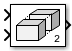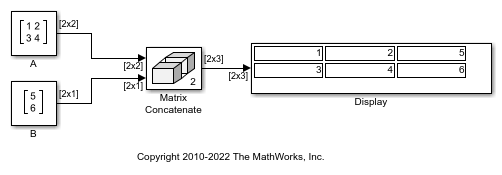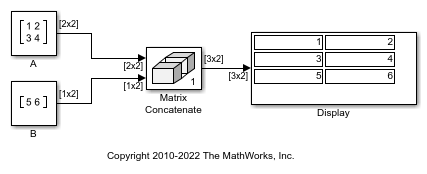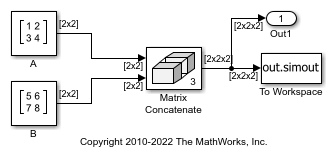# Matrix Concatenate

Concatenate input matrices of same data type for iterative processing

•Libraries:
DSP System Toolbox / Math Functions / Matrices and Linear Algebra / Matrix Operations
HDL Coder / Math Operations

Alternative Configurations of Matrix Concatenate Block:
Vector Concatenate

## Description

The Matrix Concatenate block concatenates input signals to create a nonscalar signal that you can iteratively process with a subsystem, for example, a for-each, while-iterator, or for-iterator subsystem.

You can use multiple Matrix Concatenate blocks to create the output signal in stages, but the result is flat along each concatenation dimension, as if you used a single block to concatenate the signals.

The signals in the output signal appear in the same order as the input signals for the block along the concatenation dimension. For a description of the port order for various block orientations, see Identify Port Location on Rotated or Flipped Block.

You must use a Vector Concatenate or Matrix Concatenate block to define an array of buses. For more information, see Group Nonvirtual Buses in Arrays of Buses.

## Examples

expand all

When a Matrix Concatenate block receives 2-D matrices with the same number of rows, you can horizontally concatenate the matrices, placing them side-by-side in the output matrix. To horizontally concatenate the matrices, set the Concatenate dimension block parameter to `2`.

For example, simulate the `ex_concatenate_horizontal` model.The input matrices [1 2;3 4] and [5;6] are horizontally concatenated to create the output matrix [1 2 5;3 4 6].

When a Matrix Concatenate block receives 2-D matrices with the same number of columns, you can vertically concatenate the matrices, placing them on top of each other in the output matrix. To vertically concatenate the matrices, set the Concatenate dimension block parameter to `1`.

For example, simulate the `ex_concatenate_vertical` model.The input matrices [1 2;3 4] and [5 6] are vertically concatenated to create the output matrix [1 2;3 4;5 6].

When a Matrix Concatenate block receives 2-D matrices, you can perform multidimensional matrix concatenation. To do so, set the Concatenate dimension block parameter to `3`.

For example, simulate the `ex_concatenate_multidims` model.The dimension of each input matrix is [2x2], and the dimension of the output matrix is [2x2x2].

## Ports

### Input

expand all

First input to concatenate, specified as a scalar, vector, matrix, or array.

• Inputs must be of the same data type.

• Matrix and array inputs are supported only when you set Mode to ```Multidimensional array```.

When the data type is a `Simulink.Bus` object, the inputs must be nonvirtual buses.

Data Types: `single` | `double` | `half` | `int8` | `int16` | `int32` | `int64` | `uint8` | `uint16` | `uint32` | `uint64` | `Boolean` | `fixed point` | `enumerated` | `bus` | `image`

Nth input to concatenate, specified as a scalar, vector, matrix, or array.

• Inputs must be of the same data type.

• Matrix and array inputs are supported only when you set Mode to ```Multidimensional array```.

#### Dependencies

To add input ports, set Number of inputs to an integer greater than or equal to 2.

Data Types: `single` | `double` | `half` | `int8` | `int16` | `int32` | `int64` | `uint8` | `uint16` | `uint32` | `uint64` | `Boolean` | `fixed point` | `enumerated` | `bus` | `image`

### Output

expand all

Concatenation of input signals along specified dimension. Outputs have the same data type as the input.

Data Types: `single` | `double` | `half` | `int8` | `int16` | `int32` | `int64` | `uint8` | `uint16` | `uint32` | `uint64` | `Boolean` | `fixed point` | `enumerated` | `bus` | `image`

## Parameters

expand all

To edit block parameters interactively, use the Property Inspector. From the Simulink® Toolstrip, on the Simulation tab, in the Prepare gallery, select .

Specify the number of inputs for the block as a real-valued, positive integer, less than or equal to 65536.

#### Programmatic Use

To set the block parameter value programmatically, use the `set_param` function.

 Parameter: `NumInputs` Values: `'2'` (default) | positive integer in quotes Data Types: `char` | `string`

Example: `set_param(gcb,'NumInputs','3')`

Select whether the block operates in vector or multidimensional array concatenation mode. The default Mode of the Vector Concatenate block is `Vector`. The default Mode of the Matrix Concatenate block is `Multidimensional array`.

• When you select `Vector`, the block performs vector concatenation.

• When you select `Multidimensional array`, the block performs matrix concatenation.

Mode SettingInput SignalsOutput Signal
`Vector`

• Vectors

• Row vectors (1-by-M matrices)

• Column vectors (M-by-1 matrices)

• Combination of vectors and either row or column vectors

When all inputs are vectors, the output is a vector.

If any of the inputs are row or column vectors, the output is a row or column vector, respectively.

`Multidimensional array`

Signals of any dimensionality (scalars, vectors, and matrices)

The output is always an array.

Trailing dimensions are assumed to be `1` for lower dimensionality inputs. For example, if the output is 4-D and the input is `[2x3]` (2-D), this block treats the input as `[2x3x1x1]`.

Concatenation is on the dimension that you specify with the Concatenate dimension parameter.

#### Programmatic Use

To set the block parameter value programmatically, use the `set_param` function.

 Parameter: `Mode` Values: `'Vector'` | `'Multidimensional array'`

Example: `set_param(gcb,'Mode','Vector')`

Specify the output dimension along which to concatenate the input arrays.

• `1` — Concatenate inputs vertically. The vertical matrix concatenation stacks the input matrices on top of each other in the output matrix. When you insert a Vector Concatenate block and set Mode to `Multidimensional array`, the default is `1`.

• `2` — Concatenate inputs horizontally. The horizontal matrix concatenation places the input matrices side-by-side in the output matrix. When you insert a Matrix Concatenate block, the default is `2`.

• `3` or more — Perform multidimensional concatenation on the inputs.

The input matrices must have compatible sizes for concatenation. Vertical concatenation requires the input matrices to have the same number of columns. Horizontal concatenation requires input matrices to have the same number of rows.

#### Dependencies

To enable this parameter, set Mode to `Multidimensional array`.

#### Programmatic Use

To set the block parameter value programmatically, use the `set_param` function.

 Parameter: `ConcatenateDimension` Values: scalar integer in quotes Data Types: `char` | `string`

Example: `set_param(gcb,'ConcatenateDimension','2')`

## Block Characteristics

 Data Types `Boolean` | `double` | `enumerated` | `fixed point` | `half` | `image` | `integer` | `single` Direct Feedthrough `yes` Multidimensional Signals `yes` Variable-Size Signals `yes` Zero-Crossing Detection `no`

## Alternative Configurations

expand all

The Vector Concatenate block sets Mode to `Vector`.

Libraries:
HDL Coder / Math Operations
HDL Coder / Signal Routing

## Version History

Introduced in R2009b